# Fisseha Berhane, PhD

## Improving Deep Neural Networks: Initialization¶

Welcome to the first assignment of "Improving Deep Neural Networks".

Training your neural network requires specifying an initial value of the weights. A well chosen initialization method will help learning.

If you completed the previous course of this specialization, you probably followed our instructions for weight initialization, and it has worked out so far. But how do you choose the initialization for a new neural network? In this notebook, you will see how different initializations lead to different results.

A well chosen initialization can:

• Speed up the convergence of gradient descent
• Increase the odds of gradient descent converging to a lower training (and generalization) error

To get started, run the following cell to load the packages and the planar dataset you will try to classify.

In :
import numpy as np
import matplotlib.pyplot as plt
import sklearn
import sklearn.datasets
from init_utils import sigmoid, relu, compute_loss, forward_propagation, backward_propagation
from init_utils import update_parameters, predict, load_dataset, plot_decision_boundary, predict_dec

%matplotlib inline
plt.rcParams['figure.figsize'] = (7.0, 4.0) # set default size of plots
plt.rcParams['image.interpolation'] = 'nearest'
plt.rcParams['image.cmap'] = 'gray'

# load image dataset: blue/red dots in circles
train_X, train_Y, test_X, test_Y = load_dataset()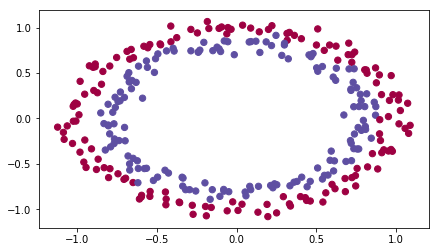You would like a classifier to separate the blue dots from the red dots.

## 1 - Neural Network model¶

You will use a 3-layer neural network (already implemented for you). Here are the initialization methods you will experiment with:

• Zeros initialization -- setting initialization = "zeros" in the input argument.
• Random initialization -- setting initialization = "random" in the input argument. This initializes the weights to large random values.
• He initialization -- setting initialization = "he" in the input argument. This initializes the weights to random values scaled according to a paper by He et al., 2015.

Instructions: Please quickly read over the code below, and run it. In the next part you will implement the three initialization methods that this model() calls.

In :
def model(X, Y, learning_rate = 0.01, num_iterations = 15000, print_cost = True, initialization = "he"):
"""
Implements a three-layer neural network: LINEAR->RELU->LINEAR->RELU->LINEAR->SIGMOID.

Arguments:
X -- input data, of shape (2, number of examples)
Y -- true "label" vector (containing 0 for red dots; 1 for blue dots), of shape (1, number of examples)
learning_rate -- learning rate for gradient descent
num_iterations -- number of iterations to run gradient descent
print_cost -- if True, print the cost every 1000 iterations
initialization -- flag to choose which initialization to use ("zeros","random" or "he")

Returns:
parameters -- parameters learnt by the model
"""

costs = [] # to keep track of the loss
m = X.shape # number of examples
layers_dims = [X.shape, 10, 5, 1]

# Initialize parameters dictionary.
if initialization == "zeros":
parameters = initialize_parameters_zeros(layers_dims)
elif initialization == "random":
parameters = initialize_parameters_random(layers_dims)
elif initialization == "he":
parameters = initialize_parameters_he(layers_dims)

for i in range(0, num_iterations):

# Forward propagation: LINEAR -> RELU -> LINEAR -> RELU -> LINEAR -> SIGMOID.
a3, cache = forward_propagation(X, parameters)

# Loss
cost = compute_loss(a3, Y)

# Backward propagation.

# Update parameters.

# Print the loss every 1000 iterations
if print_cost and i % 1000 == 0:
print("Cost after iteration {}: {}".format(i, cost))
costs.append(cost)

# plot the loss
plt.plot(costs)
plt.ylabel('cost')
plt.xlabel('iterations (per hundreds)')
plt.title("Learning rate =" + str(learning_rate))
plt.show()

return parameters


## 2 - Zero initialization¶

There are two types of parameters to initialize in a neural network:

• the weight matrices $(W^{}, W^{}, W^{}, ..., W^{[L-1]}, W^{[L]})$
• the bias vectors $(b^{}, b^{}, b^{}, ..., b^{[L-1]}, b^{[L]})$

Exercise: Implement the following function to initialize all parameters to zeros. You'll see later that this does not work well since it fails to "break symmetry", but lets try it anyway and see what happens. Use np.zeros((..,..)) with the correct shapes.

In :
# GRADED FUNCTION: initialize_parameters_zeros

def initialize_parameters_zeros(layers_dims):
"""
Arguments:
layer_dims -- python array (list) containing the size of each layer.

Returns:
parameters -- python dictionary containing your parameters "W1", "b1", ..., "WL", "bL":
W1 -- weight matrix of shape (layers_dims, layers_dims)
b1 -- bias vector of shape (layers_dims, 1)
...
WL -- weight matrix of shape (layers_dims[L], layers_dims[L-1])
bL -- bias vector of shape (layers_dims[L], 1)
"""

parameters = {}
L = len(layers_dims)            # number of layers in the network

for l in range(1, L):
### START CODE HERE ### (≈ 2 lines of code)
parameters['W' + str(l)] = np.zeros((layers_dims[l], layers_dims[l-1]))
parameters['b' + str(l)] = np.zeros((layers_dims[l], 1))
### END CODE HERE ###
return parameters

In :
parameters = initialize_parameters_zeros([3,2,1])
print("W1 = " + str(parameters["W1"]))
print("b1 = " + str(parameters["b1"]))
print("W2 = " + str(parameters["W2"]))
print("b2 = " + str(parameters["b2"]))

W1 = [[ 0.  0.  0.]
[ 0.  0.  0.]]
b1 = [[ 0.]
[ 0.]]
W2 = [[ 0.  0.]]
b2 = [[ 0.]]


Expected Output:

 **W1** [[ 0. 0. 0.] [ 0. 0. 0.]] **b1** [[ 0.] [ 0.]] **W2** [[ 0. 0.]] **b2** [[ 0.]]

Run the following code to train your model on 15,000 iterations using zeros initialization.

In :
parameters = model(train_X, train_Y, initialization = "zeros")
print ("On the train set:")
predictions_train = predict(train_X, train_Y, parameters)
print ("On the test set:")
predictions_test = predict(test_X, test_Y, parameters)

Cost after iteration 0: 0.6931471805599453
Cost after iteration 1000: 0.6931471805599453
Cost after iteration 2000: 0.6931471805599453
Cost after iteration 3000: 0.6931471805599453
Cost after iteration 4000: 0.6931471805599453
Cost after iteration 5000: 0.6931471805599453
Cost after iteration 6000: 0.6931471805599453
Cost after iteration 7000: 0.6931471805599453
Cost after iteration 8000: 0.6931471805599453
Cost after iteration 9000: 0.6931471805599453
Cost after iteration 10000: 0.6931471805599455
Cost after iteration 11000: 0.6931471805599453
Cost after iteration 12000: 0.6931471805599453
Cost after iteration 13000: 0.6931471805599453
Cost after iteration 14000: 0.6931471805599453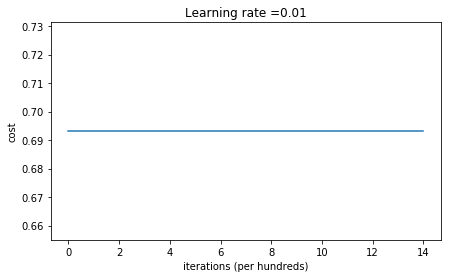On the train set:
Accuracy: 0.5
On the test set:
Accuracy: 0.5


The performance is really bad, and the cost does not really decrease, and the algorithm performs no better than random guessing. Why? Lets look at the details of the predictions and the decision boundary:

In :
print ("predictions_train = " + str(predictions_train))
print ("predictions_test = " + str(predictions_test))

predictions_train = [[0 0 0 0 0 0 0 0 0 0 0 0 0 0 0 0 0 0 0 0 0 0 0 0 0 0 0 0 0 0 0 0 0 0 0 0 0
0 0 0 0 0 0 0 0 0 0 0 0 0 0 0 0 0 0 0 0 0 0 0 0 0 0 0 0 0 0 0 0 0 0 0 0 0
0 0 0 0 0 0 0 0 0 0 0 0 0 0 0 0 0 0 0 0 0 0 0 0 0 0 0 0 0 0 0 0 0 0 0 0 0
0 0 0 0 0 0 0 0 0 0 0 0 0 0 0 0 0 0 0 0 0 0 0 0 0 0 0 0 0 0 0 0 0 0 0 0 0
0 0 0 0 0 0 0 0 0 0 0 0 0 0 0 0 0 0 0 0 0 0 0 0 0 0 0 0 0 0 0 0 0 0 0 0 0
0 0 0 0 0 0 0 0 0 0 0 0 0 0 0 0 0 0 0 0 0 0 0 0 0 0 0 0 0 0 0 0 0 0 0 0 0
0 0 0 0 0 0 0 0 0 0 0 0 0 0 0 0 0 0 0 0 0 0 0 0 0 0 0 0 0 0 0 0 0 0 0 0 0
0 0 0 0 0 0 0 0 0 0 0 0 0 0 0 0 0 0 0 0 0 0 0 0 0 0 0 0 0 0 0 0 0 0 0 0 0
0 0 0 0]]
predictions_test = [[0 0 0 0 0 0 0 0 0 0 0 0 0 0 0 0 0 0 0 0 0 0 0 0 0 0 0 0 0 0 0 0 0 0 0 0 0
0 0 0 0 0 0 0 0 0 0 0 0 0 0 0 0 0 0 0 0 0 0 0 0 0 0 0 0 0 0 0 0 0 0 0 0 0
0 0 0 0 0 0 0 0 0 0 0 0 0 0 0 0 0 0 0 0 0 0 0 0 0 0]]

In :
plt.title("Model with Zeros initialization")
axes = plt.gca()
axes.set_xlim([-1.5,1.5])
axes.set_ylim([-1.5,1.5])
plot_decision_boundary(lambda x: predict_dec(parameters, x.T), train_X, train_Y)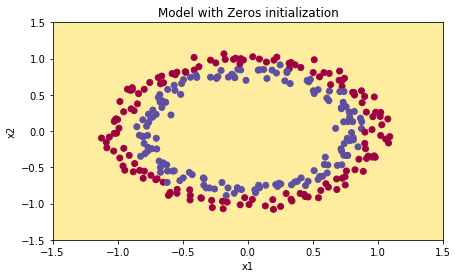The model is predicting 0 for every example.

In general, initializing all the weights to zero results in the network failing to break symmetry. This means that every neuron in each layer will learn the same thing, and you might as well be training a neural network with $n^{[l]}=1$ for every layer, and the network is no more powerful than a linear classifier such as logistic regression.

What you should remember:

• The weights $W^{[l]}$ should be initialized randomly to break symmetry.
• It is however okay to initialize the biases $b^{[l]}$ to zeros. Symmetry is still broken so long as $W^{[l]}$ is initialized randomly.

## 3 - Random initialization¶

To break symmetry, lets intialize the weights randomly. Following random initialization, each neuron can then proceed to learn a different function of its inputs. In this exercise, you will see what happens if the weights are intialized randomly, but to very large values.

Exercise: Implement the following function to initialize your weights to large random values (scaled by *10) and your biases to zeros. Use np.random.randn(..,..) * 10 for weights and np.zeros((.., ..)) for biases. We are using a fixed np.random.seed(..) to make sure your "random" weights match ours, so don't worry if running several times your code gives you always the same initial values for the parameters.

In :
# GRADED FUNCTION: initialize_parameters_random

def initialize_parameters_random(layers_dims):
"""
Arguments:
layer_dims -- python array (list) containing the size of each layer.

Returns:
parameters -- python dictionary containing your parameters "W1", "b1", ..., "WL", "bL":
W1 -- weight matrix of shape (layers_dims, layers_dims)
b1 -- bias vector of shape (layers_dims, 1)
...
WL -- weight matrix of shape (layers_dims[L], layers_dims[L-1])
bL -- bias vector of shape (layers_dims[L], 1)
"""

np.random.seed(3)               # This seed makes sure your "random" numbers will be the as ours
parameters = {}
L = len(layers_dims)            # integer representing the number of layers

for l in range(1, L):
### START CODE HERE ### (≈ 2 lines of code)
parameters['W' + str(l)] = np.random.randn(layers_dims[l], layers_dims[l-1]) * 10
parameters['b' + str(l)] = np.zeros((layers_dims[l], 1))
### END CODE HERE ###

return parameters

In :
parameters = initialize_parameters_random([3, 2, 1])
print("W1 = " + str(parameters["W1"]))
print("b1 = " + str(parameters["b1"]))
print("W2 = " + str(parameters["W2"]))
print("b2 = " + str(parameters["b2"]))

W1 = [[ 17.88628473   4.36509851   0.96497468]
[-18.63492703  -2.77388203  -3.54758979]]
b1 = [[ 0.]
[ 0.]]
W2 = [[-0.82741481 -6.27000677]]
b2 = [[ 0.]]


Expected Output:

 **W1** [[ 17.88628473 4.36509851 0.96497468] [-18.63492703 -2.77388203 -3.54758979]] **b1** [[ 0.] [ 0.]] **W2** [[-0.82741481 -6.27000677]] **b2** [[ 0.]]

Run the following code to train your model on 15,000 iterations using random initialization.

In :
parameters = model(train_X, train_Y, initialization = "random")
print ("On the train set:")
predictions_train = predict(train_X, train_Y, parameters)
print ("On the test set:")
predictions_test = predict(test_X, test_Y, parameters)

/home/jovyan/work/week5/Initialization/init_utils.py:145: RuntimeWarning: divide by zero encountered in log
logprobs = np.multiply(-np.log(a3),Y) + np.multiply(-np.log(1 - a3), 1 - Y)
/home/jovyan/work/week5/Initialization/init_utils.py:145: RuntimeWarning: invalid value encountered in multiply
logprobs = np.multiply(-np.log(a3),Y) + np.multiply(-np.log(1 - a3), 1 - Y)

Cost after iteration 0: inf
Cost after iteration 1000: 0.6237287551108738
Cost after iteration 2000: 0.5981106708339466
Cost after iteration 3000: 0.5638353726276827
Cost after iteration 4000: 0.550152614449184
Cost after iteration 5000: 0.5444235275228304
Cost after iteration 6000: 0.5374184054630083
Cost after iteration 7000: 0.47357131493578297
Cost after iteration 8000: 0.39775634899580387
Cost after iteration 9000: 0.3934632865981078
Cost after iteration 10000: 0.39202525076484457
Cost after iteration 11000: 0.38921493051297673
Cost after iteration 12000: 0.38614221789840486
Cost after iteration 13000: 0.38497849983013926
Cost after iteration 14000: 0.38278397192120406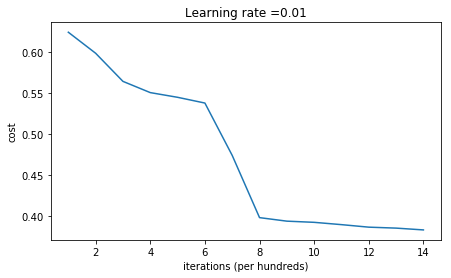On the train set:
Accuracy: 0.83
On the test set:
Accuracy: 0.86


If you see "inf" as the cost after the iteration 0, this is because of numerical roundoff; a more numerically sophisticated implementation would fix this. But this isn't worth worrying about for our purposes.

Anyway, it looks like you have broken symmetry, and this gives better results. than before. The model is no longer outputting all 0s.

In :
print (predictions_train)
print (predictions_test)

[[1 0 1 1 0 0 1 1 1 1 1 0 1 0 0 1 0 1 1 0 0 0 1 0 1 1 1 1 1 1 0 1 1 0 0 1 1
1 1 1 1 1 1 0 1 1 1 1 0 1 0 1 1 1 1 0 0 1 1 1 1 0 1 1 0 1 0 1 1 1 1 0 0 0
0 0 1 0 1 0 1 1 1 0 0 1 1 1 1 1 1 0 0 1 1 1 0 1 1 0 1 0 1 1 0 1 1 0 1 0 1
1 0 0 1 0 0 1 1 0 1 1 1 0 1 0 0 1 0 1 1 1 1 1 1 1 0 1 1 0 0 1 1 0 0 0 1 0
1 0 1 0 1 1 1 0 0 1 1 1 1 0 1 1 0 1 0 1 1 0 1 0 1 1 1 1 0 1 1 1 1 0 1 0 1
0 1 1 1 1 0 1 1 0 1 1 0 1 1 0 1 0 1 1 1 0 1 1 1 0 1 0 1 0 0 1 0 1 1 0 1 1
0 1 1 0 1 1 1 0 1 1 1 1 0 1 0 0 1 1 0 1 1 1 0 0 0 1 1 0 1 1 1 1 0 1 1 0 1
1 1 0 0 1 0 0 0 1 0 0 0 1 1 1 1 0 0 0 0 1 1 1 1 0 0 1 1 1 1 1 1 1 0 0 0 1
1 1 1 0]]
[[1 1 1 1 0 1 0 1 1 0 1 1 1 0 0 0 0 1 0 1 0 0 1 0 1 0 1 1 1 1 1 0 0 0 0 1 0
1 1 0 0 1 1 1 1 1 0 1 1 1 0 1 0 1 1 0 1 0 1 0 1 1 1 1 1 1 1 1 1 0 1 0 1 1
1 1 1 0 1 0 0 1 0 0 0 1 1 0 1 1 0 0 0 1 1 0 1 1 0 0]]

In :
plt.title("Model with large random initialization")
axes = plt.gca()
axes.set_xlim([-1.5,1.5])
axes.set_ylim([-1.5,1.5])
plot_decision_boundary(lambda x: predict_dec(parameters, x.T), train_X, train_Y)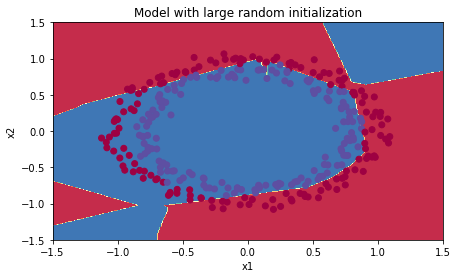Observations:

• The cost starts very high. This is because with large random-valued weights, the last activation (sigmoid) outputs results that are very close to 0 or 1 for some examples, and when it gets that example wrong it incurs a very high loss for that example. Indeed, when $\log(a^{}) = \log(0)$, the loss goes to infinity.
• Poor initialization can lead to vanishing/exploding gradients, which also slows down the optimization algorithm.
• If you train this network longer you will see better results, but initializing with overly large random numbers slows down the optimization.

In summary:

• Initializing weights to very large random values does not work well.
• Hopefully intializing with small random values does better. The important question is: how small should be these random values be? Lets find out in the next part!

## 4 - He initialization¶

Finally, try "He Initialization"; this is named for the first author of He et al., 2015. (If you have heard of "Xavier initialization", this is similar except Xavier initialization uses a scaling factor for the weights $W^{[l]}$ of sqrt(1./layers_dims[l-1]) where He initialization would use sqrt(2./layers_dims[l-1]).)

Exercise: Implement the following function to initialize your parameters with He initialization.

Hint: This function is similar to the previous initialize_parameters_random(...). The only difference is that instead of multiplying np.random.randn(..,..) by 10, you will multiply it by $\sqrt{\frac{2}{\text{dimension of the previous layer}}}$, which is what He initialization recommends for layers with a ReLU activation.

In :
# GRADED FUNCTION: initialize_parameters_he

def initialize_parameters_he(layers_dims):
"""
Arguments:
layer_dims -- python array (list) containing the size of each layer.

Returns:
parameters -- python dictionary containing your parameters "W1", "b1", ..., "WL", "bL":
W1 -- weight matrix of shape (layers_dims, layers_dims)
b1 -- bias vector of shape (layers_dims, 1)
...
WL -- weight matrix of shape (layers_dims[L], layers_dims[L-1])
bL -- bias vector of shape (layers_dims[L], 1)
"""

np.random.seed(3)
parameters = {}
L = len(layers_dims) - 1 # integer representing the number of layers

for l in range(1, L + 1):
### START CODE HERE ### (≈ 2 lines of code)
parameters['W' + str(l)] = np.random.randn(layers_dims[l], layers_dims[l-1]) * np.sqrt(2/layers_dims[l-1])
parameters['b' + str(l)] = np.zeros((layers_dims[l], 1))
### END CODE HERE ###

return parameters

In :
parameters = initialize_parameters_he([2, 4, 1])
print("W1 = " + str(parameters["W1"]))
print("b1 = " + str(parameters["b1"]))
print("W2 = " + str(parameters["W2"]))
print("b2 = " + str(parameters["b2"]))

W1 = [[ 1.78862847  0.43650985]
[ 0.09649747 -1.8634927 ]
[-0.2773882  -0.35475898]
[-0.08274148 -0.62700068]]
b1 = [[ 0.]
[ 0.]
[ 0.]
[ 0.]]
W2 = [[-0.03098412 -0.33744411 -0.92904268  0.62552248]]
b2 = [[ 0.]]


Expected Output:

 **W1** [[ 1.78862847 0.43650985] [ 0.09649747 -1.8634927 ] [-0.2773882 -0.35475898] [-0.08274148 -0.62700068]] **b1** [[ 0.] [ 0.] [ 0.] [ 0.]] **W2** [[-0.03098412 -0.33744411 -0.92904268 0.62552248]] **b2** [[ 0.]]

Run the following code to train your model on 15,000 iterations using He initialization.

In :
parameters = model(train_X, train_Y, initialization = "he")
print ("On the train set:")
predictions_train = predict(train_X, train_Y, parameters)
print ("On the test set:")
predictions_test = predict(test_X, test_Y, parameters)

Cost after iteration 0: 0.8830537463419761
Cost after iteration 1000: 0.6879825919728063
Cost after iteration 2000: 0.6751286264523371
Cost after iteration 3000: 0.6526117768893807
Cost after iteration 4000: 0.6082958970572938
Cost after iteration 5000: 0.5304944491717495
Cost after iteration 6000: 0.4138645817071794
Cost after iteration 7000: 0.3117803464844441
Cost after iteration 8000: 0.23696215330322562
Cost after iteration 9000: 0.18597287209206836
Cost after iteration 10000: 0.1501555628037182
Cost after iteration 11000: 0.12325079292273548
Cost after iteration 12000: 0.09917746546525937
Cost after iteration 13000: 0.0845705595402428
Cost after iteration 14000: 0.07357895962677366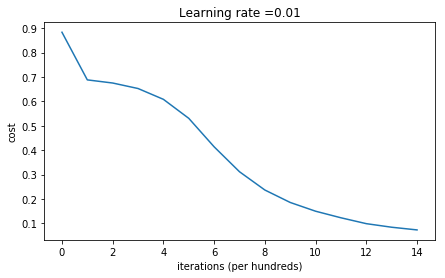On the train set:
Accuracy: 0.993333333333
On the test set:
Accuracy: 0.96

In :
plt.title("Model with He initialization")
axes = plt.gca()
axes.set_xlim([-1.5,1.5])
axes.set_ylim([-1.5,1.5])
plot_decision_boundary(lambda x: predict_dec(parameters, x.T), train_X, train_Y)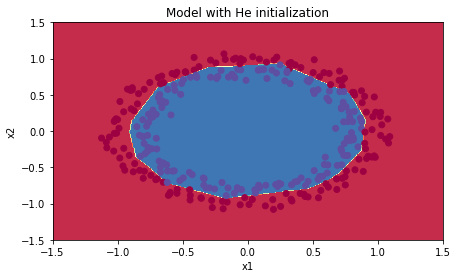Observations:

• The model with He initialization separates the blue and the red dots very well in a small number of iterations.

## 5 - Conclusions¶

You have seen three different types of initializations. For the same number of iterations and same hyperparameters the comparison is:

 **Model** **Train accuracy** **Problem/Comment** 3-layer NN with zeros initialization 50% fails to break symmetry 3-layer NN with large random initialization 83% too large weights 3-layer NN with He initialization 99% recommended method

What you should remember from this notebook:

• Different initializations lead to different results
• Random initialization is used to break symmetry and make sure different hidden units can learn different things
• Don't intialize to values that are too large
• He initialization works well for networks with ReLU activations.

### Appendix¶

code for init_utils is shown below.

In [ ]:
import numpy as np
import matplotlib.pyplot as plt
import h5py
import sklearn
import sklearn.datasets

def sigmoid(x):
"""
Compute the sigmoid of x

Arguments:
x -- A scalar or numpy array of any size.

Return:
s -- sigmoid(x)
"""
s = 1/(1+np.exp(-x))
return s

def relu(x):
"""
Compute the relu of x

Arguments:
x -- A scalar or numpy array of any size.

Return:
s -- relu(x)
"""
s = np.maximum(0,x)

return s

def forward_propagation(X, parameters):
"""
Implements the forward propagation (and computes the loss) presented in Figure 2.

Arguments:
X -- input dataset, of shape (input size, number of examples)
Y -- true "label" vector (containing 0 if cat, 1 if non-cat)
parameters -- python dictionary containing your parameters "W1", "b1", "W2", "b2", "W3", "b3":
W1 -- weight matrix of shape ()
b1 -- bias vector of shape ()
W2 -- weight matrix of shape ()
b2 -- bias vector of shape ()
W3 -- weight matrix of shape ()
b3 -- bias vector of shape ()

Returns:
loss -- the loss function (vanilla logistic loss)
"""

# retrieve parameters
W1 = parameters["W1"]
b1 = parameters["b1"]
W2 = parameters["W2"]
b2 = parameters["b2"]
W3 = parameters["W3"]
b3 = parameters["b3"]

# LINEAR -> RELU -> LINEAR -> RELU -> LINEAR -> SIGMOID
z1 = np.dot(W1, X) + b1
a1 = relu(z1)
z2 = np.dot(W2, a1) + b2
a2 = relu(z2)
z3 = np.dot(W3, a2) + b3
a3 = sigmoid(z3)

cache = (z1, a1, W1, b1, z2, a2, W2, b2, z3, a3, W3, b3)

return a3, cache

def backward_propagation(X, Y, cache):
"""
Implement the backward propagation presented in figure 2.

Arguments:
X -- input dataset, of shape (input size, number of examples)
Y -- true "label" vector (containing 0 if cat, 1 if non-cat)
cache -- cache output from forward_propagation()

Returns:
gradients -- A dictionary with the gradients with respect to each parameter, activation and pre-activation variables
"""
m = X.shape
(z1, a1, W1, b1, z2, a2, W2, b2, z3, a3, W3, b3) = cache

dz3 = 1./m * (a3 - Y)
dW3 = np.dot(dz3, a2.T)
db3 = np.sum(dz3, axis=1, keepdims = True)

da2 = np.dot(W3.T, dz3)
dz2 = np.multiply(da2, np.int64(a2 > 0))
dW2 = np.dot(dz2, a1.T)
db2 = np.sum(dz2, axis=1, keepdims = True)

da1 = np.dot(W2.T, dz2)
dz1 = np.multiply(da1, np.int64(a1 > 0))
dW1 = np.dot(dz1, X.T)
db1 = np.sum(dz1, axis=1, keepdims = True)

gradients = {"dz3": dz3, "dW3": dW3, "db3": db3,
"da2": da2, "dz2": dz2, "dW2": dW2, "db2": db2,
"da1": da1, "dz1": dz1, "dW1": dW1, "db1": db1}

"""

Arguments:
parameters -- python dictionary containing your parameters

Returns:
parameters -- python dictionary containing your updated parameters
parameters['W' + str(i)] = ...
parameters['b' + str(i)] = ...
"""

L = len(parameters) // 2 # number of layers in the neural networks

# Update rule for each parameter
for k in range(L):
parameters["W" + str(k+1)] = parameters["W" + str(k+1)] - learning_rate * grads["dW" + str(k+1)]
parameters["b" + str(k+1)] = parameters["b" + str(k+1)] - learning_rate * grads["db" + str(k+1)]

return parameters

def compute_loss(a3, Y):

"""
Implement the loss function

Arguments:
a3 -- post-activation, output of forward propagation
Y -- "true" labels vector, same shape as a3

Returns:
loss - value of the loss function
"""

m = Y.shape
logprobs = np.multiply(-np.log(a3),Y) + np.multiply(-np.log(1 - a3), 1 - Y)
loss = 1./m * np.nansum(logprobs)

return loss

train_dataset = h5py.File('datasets/train_catvnoncat.h5', "r")
train_set_x_orig = np.array(train_dataset["train_set_x"][:]) # your train set features
train_set_y_orig = np.array(train_dataset["train_set_y"][:]) # your train set labels

test_dataset = h5py.File('datasets/test_catvnoncat.h5', "r")
test_set_x_orig = np.array(test_dataset["test_set_x"][:]) # your test set features
test_set_y_orig = np.array(test_dataset["test_set_y"][:]) # your test set labels

classes = np.array(test_dataset["list_classes"][:]) # the list of classes

train_set_y = train_set_y_orig.reshape((1, train_set_y_orig.shape))
test_set_y = test_set_y_orig.reshape((1, test_set_y_orig.shape))

train_set_x_orig = train_set_x_orig.reshape(train_set_x_orig.shape, -1).T
test_set_x_orig = test_set_x_orig.reshape(test_set_x_orig.shape, -1).T

train_set_x = train_set_x_orig/255
test_set_x = test_set_x_orig/255

return train_set_x, train_set_y, test_set_x, test_set_y, classes

def predict(X, y, parameters):
"""
This function is used to predict the results of a  n-layer neural network.

Arguments:
X -- data set of examples you would like to label
parameters -- parameters of the trained model

Returns:
p -- predictions for the given dataset X
"""

m = X.shape
p = np.zeros((1,m), dtype = np.int)

# Forward propagation
a3, caches = forward_propagation(X, parameters)

# convert probas to 0/1 predictions
for i in range(0, a3.shape):
if a3[0,i] > 0.5:
p[0,i] = 1
else:
p[0,i] = 0

# print results
print("Accuracy: "  + str(np.mean((p[0,:] == y[0,:]))))

return p

def plot_decision_boundary(model, X, y):
# Set min and max values and give it some padding
x_min, x_max = X[0, :].min() - 1, X[0, :].max() + 1
y_min, y_max = X[1, :].min() - 1, X[1, :].max() + 1
h = 0.01
# Generate a grid of points with distance h between them
xx, yy = np.meshgrid(np.arange(x_min, x_max, h), np.arange(y_min, y_max, h))
# Predict the function value for the whole grid
Z = model(np.c_[xx.ravel(), yy.ravel()])
Z = Z.reshape(xx.shape)
# Plot the contour and training examples
plt.contourf(xx, yy, Z, cmap=plt.cm.Spectral)
plt.ylabel('x2')
plt.xlabel('x1')
plt.scatter(X[0, :], X[1, :], c=y, cmap=plt.cm.Spectral)
plt.show()

def predict_dec(parameters, X):
"""
Used for plotting decision boundary.

Arguments:
parameters -- python dictionary containing your parameters
X -- input data of size (m, K)

Returns
predictions -- vector of predictions of our model (red: 0 / blue: 1)
"""

# Predict using forward propagation and a classification threshold of 0.5
a3, cache = forward_propagation(X, parameters)
predictions = (a3>0.5)
return predictions